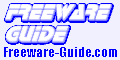Home & EducationMathematics - Page 3

Math Mechanixs
Math Mechanixs is an award winning easy to use general purpose math software program compatible with Microsoft Windows. It is ideal for students, teachers, engineers and scientists or anyone requiring an easy to use PC based mathematical software program. It will also do so much more than your calculator. Math Mechanixs was created with the belief that computers were made to solve mathematical problems.
Math Mechanixs is not a training aid or a spreadsheet program. It works using a Math Editor (as opposed to a Text Editor) allowing you to type the mathematical expressions similar to the way you would write them on a piece of paper. The software uses a multiple document interface so that you can work on multiple solutions simultaneously. There is a full feature scientific calculator for those of you who just like to press buttons. In addition there is an integrated variables and functions list window so that you can easily track your defined variables and functions.
Note: FREE registration is required after 30-day trial.

f(x)-Viewer
This program can display complex and also simple mathematical functions. Select individual colors for each graph and export the coordinate system as image. Never draw again complex curves, while you can create them as precise diagram with this program.

SAGE
Use SAGE for studying a huge range of mathematics, including algebra, calculus, elementary to very advanced number theory, cryptography, numerical computation, commutative algebra, group theory, combinatorics, graph theory, and exact linear algebra.
By using SAGE you help to support a viable open source alternative to Magma, Maple, Mathematica, and MATLAB. SAGE includes many high-quality open source math packages.
SAGE makes it easy for you to use most mathematics software together. SAGE includes interfaces to Magma, Maple, Mathematica, MATLAB, and MuPAD, and the free programs Axiom, GAP, GP/PARI, Macaulay2, Maxima, Octave, and Singular.

InftyEditor
InftyEditor is an authoring tool of mathematical documents, very easy and smooth to input mathematical expressions.
- You can input any math expressions keeping your hand on the HOME Position of your keyboard.
- The input math expressions are displayed immediately in the form of printing style as you usually see in books.
- InftyEditor has a strong Online Help of command names or syntax to input math expressions based on LaTeX. Thus, InftyEditor can be used also as a Learning Tool of LaTeX, for the beginners of LaTeX.
- The documents written in InftyEditor can be saved in different formats: XML of InftyEditor, LaTeX, MathML, Human Readable TeX for the blinds, PDF, etc.
- If InftyReader is installed in your PC, you can input texts and math expressions from printed document via scanner into InftyEditor directly.

nPlot
nPlot (pronounced 'nanoplot') aims to be a minimalistic and easy-to-use data analysing software solution.
Features:
- data visualisation (with error bars)
- linear regression (with computing coefficients errors and correlation coefficient)
- fittings: polynomial, exponential, logarithmic, power, gaussian
- peak seeking (basic)
- plot exporting to various formats: PS, EPS, SVG, PDF and PNG
- ASCII import/export.

dimensional analysis tool
I wrote a program that computes the dimensions (M, L, T, t) for an equation. I found this program extremely useful when doing derivations of formulae, since keeping the dimensions of the LHS and the RHS in synchronization is essential. You can check the dimensions of any expression by dragging and dropping units onto the work area. You can also check the dimensions of just the numerator or just the denominator.Home & Education
Calculators
Calendars & Planners
Chemistry
Food & Beverage
Genealogy
Geography
Health & Nutrition
Hobbies
Home Inventory
Kids & Parenting
Language
Mathematics
Miscellaneous
Religion & Spirituality
Science
Teaching Tools

© The Freeware Guide